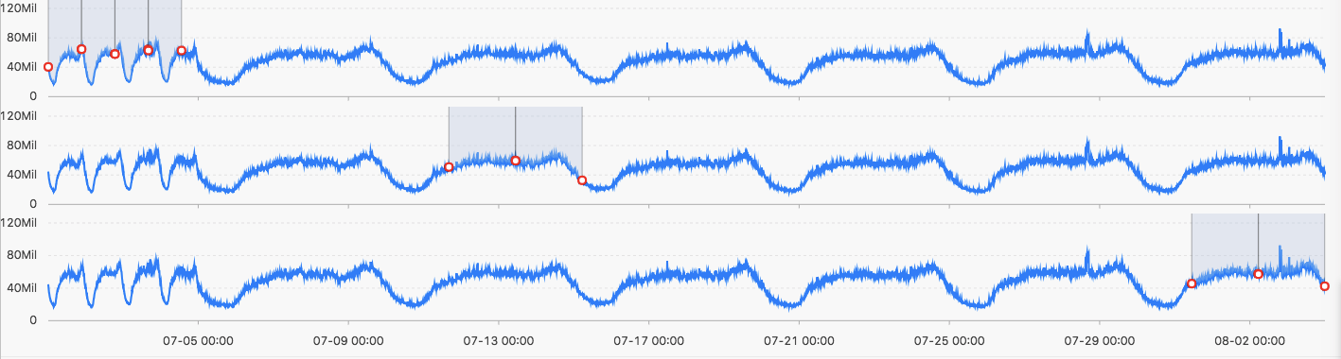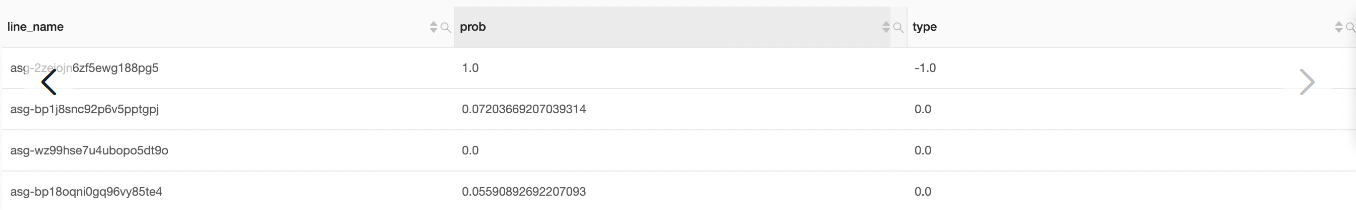This topic describes multi-period estimation functions that you can use to estimate the periodicity of time series data distributed in different time intervals and extract the periodicity by using a series of operations such as Fourier transform (FT).

## Function list

Function Description
ts_period_detect Estimates the periodicity of time series data distributed in different time intervals.
ts_period_classify Uses FT to calculate the periodicity of specified time series curves. This function can be used to identify periodic curves.

## ts_period_detect

Function format:
``select ts_period_detect(x,y,minPeriod,maxPeriod)``
The following table lists the parameters of the function format.
Parameter Description Value
x The time sequence. Points in time are sorted in ascending order along the horizontal axis. Each point in time is a Unix timestamp. Unit: seconds.
y The sequence of numeric data corresponding to each specified point in time. N/A.
minPeriod The ratio of the length to estimate to the total length of values on the time series curve. The parameter value must be a decimal number. Valid values: (0.0, 1.0].
maxPeriod The ratio of the length to estimate to the total length of values on the time series curve.
Note The maxPeriod parameter value must be greater than that of the minPeriod parameter.
The parameter value must be a decimal number. Valid values: (0.0, 1.0].
Example:
• The query statement is as follows:
``* | select ts_period_detect(stamp, value, 0.2, 1.0) from ( select __time__ - __time__ % 120 as stamp, avg(v) as value from log GROUP BY stamp order by stamp )``
• Output resultThe following table lists the display items.
Display item Description
period_id The number of the period, which is an array that includes a single value. If the length is set to 0, the original time series curve is used.
time_series The sequence of timestamps.
data_series The sequence of data corresponding to each timestamp.
• If period_id is set to 0, the result is returned based on the original time series curve.
• If period_id is set to a value other than 0, the result is returned based on the selected value.

## ts_period_classify

Function format:
``select ts_period_classify(stamp,value,instanceName)``
The following table lists the parameters of the function format.
Parameter Description Value
stamp The time sequence. Points in time are sorted in ascending order along the horizontal axis. Each point in time is a Unix timestamp. Unit: seconds.
value The sequence of numeric data corresponding to each specified point in time. N/A.
instanceName The name of the time series curve. N/A..
Example:
• The query statement is as follows:
``* and h : nu2h05202.nu8 | select ts_period_classify(stamp, value, name) from log``
• Output resultThe following table lists the display items.
Display item Description
line_name The name of the time series curve.
prob The ratio of the number of values within the primary period to the total number of values on the time series curve. Valid values: [0, 1]. You can set the value to 0.15 for testing.
type The type of the time series curve. Valid values: -1, -2, and 0.
• A value of -1 indicates that the length of values on the time series curve is too short (smaller than 64).
• A value of -2 indicates the time series curve has a high failure rate that exceeds 20%.
• A value of 0 indicates the time series curve has significant periodicity.